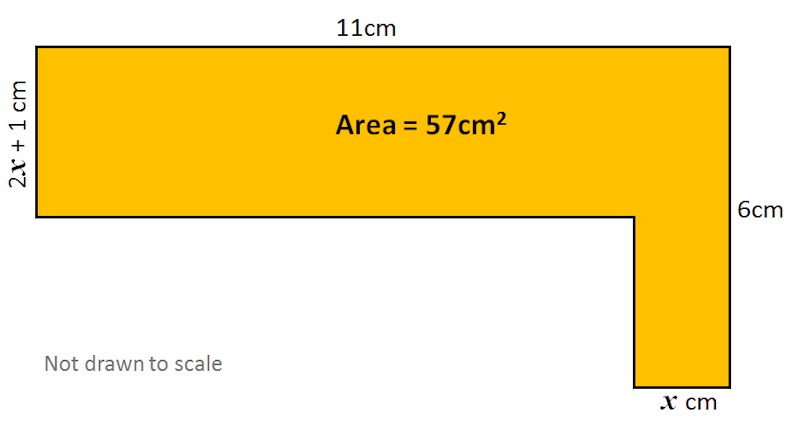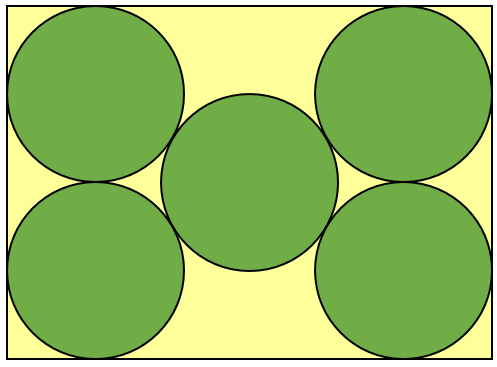# Exam-Style Questions.

## Problems adapted from questions set for previous Mathematics exams.

### 1.

GCSE Higher

The diagram below shows two rectangles not drawn to scale.

The perimeter of rectangle EFGH is 41cm and the area of rectangle ABCD is 55cm2.

• EF = 9.5cm
• AB = FG### 2.

GCSE HigherFind the value of $$x$$.

### 3.

GCSE Higher

Find the length of the sides of a square from the following clues:

• The square is drawn inside a circle.
• Each vertex of the square lies on the circumference of the circle.
• The area of the circle is 64cm2.

### 4.

GCSE Higher

Five identical circles fit exactly inside a rectangle as shown in the diagram.

Find the area of the rectangle in terms of $$r$$, the radius of the circles.### 5.

GCSE Higher

Two identical small yellow circles are drawn inside one large circle, as shown in the diagram. The centres of the small circles lie on the diameter of the large circle. The part of the large circle that is outside both small circles is painted red.Find the fraction of the large circle that is painted red.

If you would like space on the right of the question to write out the solution try this Thinning Feature. It will collapse the text into the left half of your screen but large diagrams will remain unchanged.

The exam-style questions appearing on this site are based on those set in previous examinations (or sample assessment papers for future examinations) by the major examination boards. The wording, diagrams and figures used in these questions have been changed from the originals so that students can have fresh, relevant problem solving practice even if they have previously worked through the related exam paper.

The solutions to the questions on this website are only available to those who have a Transum Subscription.

Exam-Style Questions Main Page

Search for exam-style questions containing a particular word or phrase:

To search the entire Transum website use the search box in the grey area below.